# Arduino Accelerometer mma7361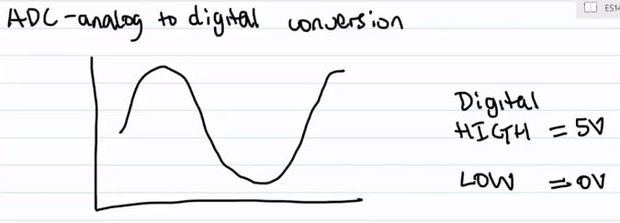Hey guys, I am a teaching assistant for an introduction to engineering class for biomedical engineering majors at Vanderbilt University this semester. I created this video to explain analog-to-digital conversion to them because time ran out during class and I did not get to this point in the lecture. I ran across the Teach It! Instructables contest and thought if I already had the video created, why not enter it into the contest, so here goes.

## Analog to Digital Conversion Tutorial

The video gives a simple introduction to analog-to-digital conversion and then explains how this relates to reading data from an accelerometer using an Arduino. For those of you who do not know, as the name suggests, an accelerometer measures the gravitational pull on the device. This particularly accelerometer measures acceleration in the x, y, and z axes. The accelerometer I am using in the demo is the MMA7361 and the datasheet can be found online. The datasheet will give more in-depth info of the accelerometer itself. If you search “MMA7361 filetype:pdf” in Google, it should pop right up. It is also attached in this Instructable. If you are not used to reading datasheets, it may be a little intimidating. Please feel free to ask if you have any questions. Additionally, the accelerometer module that I am using was purchased on Amazon from Virtuabotix, if you are interested. Anyways, here’s my video. The video itself is self-sufficient, but I highlighted the major parts of it in steps if you want a quick summary. I hope you learn something from it. And if you have any questions, please feel free to ask.

If you like my Instructable, please consider voting for it in the Instructables Teach It! Contest.

FKNARWGI0DOGDWO(1)

toturiol

## Step 1: What is Analog-to-Digital ConversionAnalog-to-digital conversion (ADC) is the process is taking a variable signal and “digitizing” the signal so that a computer can process it.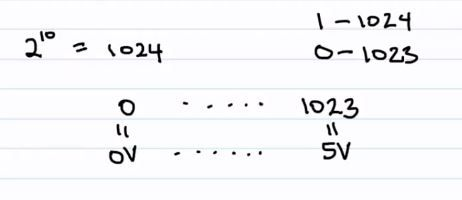An Arduino has a 10-bit ADC, meaning that the voltages that the Arduino is reading from some sensor (in our case the sensor is an accelerometer) is represented by a number in the range of 0-1023. The max voltage an Arduino reads is 5 V and the smallest is 0 V. These voltages are represented by 1023 and 0 respectively.

A discussion on bits can get a little more extensive and a little outside the scope of this Instructable, so feel free to explore this a little more on your own or ask me in the comments section.

## Step 3: Converting from Voltage to ADC Output and Vice-Versa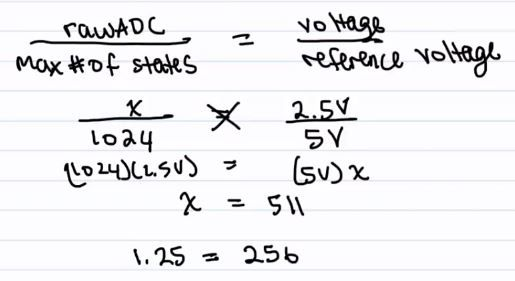If you are reading a voltage of 2.5 V, you can calculate the Arduino’s ADC output by doing a simple proportion. Oftentimes, you are reading an unknown voltage and want to use the Arduino’s ADC output to determine what voltage you are sensing. Simply modify the proportion accordingly.

## Step 4: Understanding AccelerometersWe can use an Arduino to sense the voltage outputted by an accelerometer. This voltage corresponds to an acceleration.

## Step 5: Accelerometer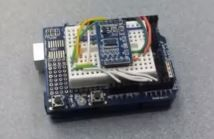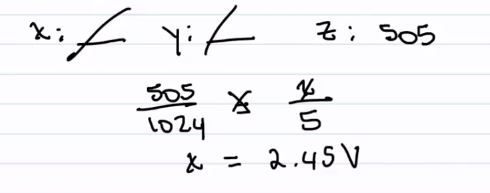If we have the accelerometer top-side up, these are the values we can expect to get from the Arduino’s ADC.

Sorry I used “x” as my variable in this example. We are calculating the acceleration in the “z-axis.” Using “x” as my variable is a habit. “x” was the first variable of choice in my Algebra classes.

## Step 6: Accelerometer Bottom-Side Up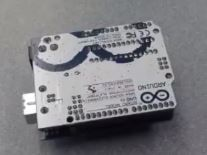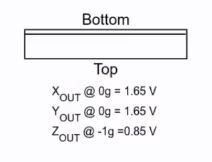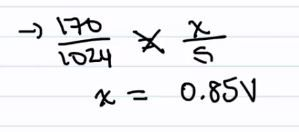If we have the accelerometer bottom-side up (z-axis down), these are the values we could expect.

Again, we are calculating acceleration in the z-axis not “x.”